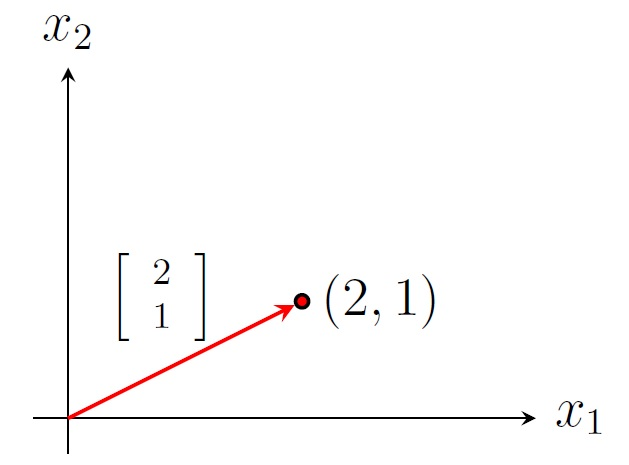Numerical Analysis Home

## Introduction to Linear Algebra

Matrix: An $$m\times n$$ matrix $$A$$ is an $$m$$-by-$$n$$ array of scalars from a field (for example real numbers) of the form $A=\left[\begin{array}{cccc} a_{11}&a_{12}&\cdots &a_{1n}\\ a_{21}&a_{22}&\cdots &a_{2n}\\ \vdots&\vdots&\ddots &\vdots\\ a_{m1}&a_{m2}&\cdots &a_{mn} \end{array}\right].$ The order (or size) of $$A$$ is $$m\times n$$ (read as m by n) if $$A$$ has $$m$$ rows and $$n$$ columns. The $$(i,j)$$-entry of $$A=[a_{i,j}]$$ is $$a_{i,j}$$.

For example, $$A=\left[\begin{array}{rrr}1&2&0\\-3&0&-1\end{array} \right]$$ is a $$2\times 3$$ real matrix. The $$(2,3)$$-entry of $$A$$ is $$-1$$.

Useful Matrices:

• A zero matrix, denoted by $$O$$ or $$O_{m,n}$$, is an $$m\times n$$ matrix whose all entries are zero.

• A square matrix is a matrix is a matrix whose number of rows and number of columns are the same.

• A diagonal matrix is a square $$n\times n$$ matrix whose nondiagonal entries are zero.

• The identity matrix of order $$n$$, denoted by $$I_n$$, is the $$n\times n$$ diagonal matrix whose diagonal entries are 1. For example, $$I_3=\left[\begin{array}{rrr}1&0&0\\0&1&0\\0&0&1 \end{array} \right]$$ is the $$3\times 3$$ identity matrix.

• An $$n\times 1$$ matrix is called a column matrix or an $$n$$-dimensional (column) vector, denoted by lowercase letters such $$x$$, $$\boldsymbol x$$, or $$\overrightarrow{x}$$. For example, $$\overrightarrow{x}=\left[\begin{array}{r}0\\1\\2 \end{array} \right]$$ is a $$3$$-dimensional vector which represents the position vector of the point $$(0,1,2)$$ in the 3-space $$\mathbb R^3$$ (i.e., a directed line segment from the origin $$(0,0,0)$$ to the point $$(0,1,2)$$).Position vector of a point in the 2-space $$\mathbb R^2$$

Matrix Operations:

Norm: We will use only two norms of a vector $$\overrightarrow{x}=\left[\begin{array}{c}x_1\\\vdots\\x_n \end{array} \right]$$:

1. $$l_2$$ norm (Euclidean norm): $$||\overrightarrow{x}||_2=\sqrt{x_1^2+\cdots+ x_n^2}$$

2. $$l_{\infty}$$ norm: $$||\overrightarrow{x}||_{\infty}=\displaystyle\max_{1\leq i\leq n} |x_i|$$

For $$\overrightarrow{x}=[-2,0,1]^T$$, $$||\overrightarrow{x}||_2=\sqrt{5}$$ and $$||\overrightarrow{x}||_{\infty}=2$$. Note that $$||\overrightarrow{x}||_{\infty}\leq ||\overrightarrow{x}||_2$$ for all $$\overrightarrow{x}$$.

Transpose: The transpose of an $$m\times n$$ matrix $$A$$, denoted by $$A^T$$, is an $$n\times m$$ matrix whose columns are corresponding rows of $$A$$, i.e., $$(A^T)_{ij}=A_{ji}$$.
Example. If $$A=\left[\begin{array}{rrr}1&2&0\\-3&0&-1\end{array} \right]$$, then $$A^T=\left[\begin{array}{rr}1&-3\\2&0\\0&-1\end{array} \right]$$.

Scalar Multiplication: Let $$A$$ be a matrix and $$c$$ be a scalar. The scalar multiple, denoted by $$cA$$, is the matrix whose entries are $$c$$ times the corresponding entries of $$A$$.
Example. If $$A=\left[\begin{array}{rrr}1&2&0\\-3&0&-1\end{array} \right]$$, then $$-2A=\left[\begin{array}{rrr}-2&-4&0\\6&0&2\end{array} \right]$$.

Sum: If $$A$$ and $$B$$ are $$m\times n$$ matrices, then the sum $$A+B$$ is the $$m\times n$$ matrix whose entries are the sum of the corresponding entries of $$A$$ and $$B$$, i.e., $$(A+B)_{ij}=A_{ij}+B_{ij}$$.
Example. If $$A=\left[\begin{array}{rrr}1&2&0\\-3&0&-1\end{array} \right]$$ and $$B=\left[\begin{array}{rrr}0&-2&0\\3&0&2\end{array} \right]$$, then $$A+B=\left[\begin{array}{rrr}1&0&0\\0&0&1\end{array} \right]$$.

Multiplication:
Matrix-vector multiplication: If $$A$$ is an $$m\times n$$ matrix and $$\overrightarrow{x}$$ is an $$n$$-dimensional vector, then their product $$A\overrightarrow{x}$$ is an $$n$$-dimensional vector whose $$(i,1)$$-entry is $$a_{i1}x_1+a_{i2}x_2+\cdots+a_{im}x_n$$, the dot product of the row $$i$$ of $$A$$ and $$\overrightarrow{x}$$. Note that $A\overrightarrow{x}=\left[\begin{array}{c} a_{11}x_1+a_{12}x_2+\cdots+a_{1n}x_n\\ a_{21}x_1+a_{22}x_2+\cdots+a_{2n}x_n\\ \vdots\\ a_{m1}x_1+a_{m2}x_2+\cdots+a_{mn}x_n\end{array}\right] = x_1\left[\begin{array}{c} a_{11}\\ a_{21}\\ \vdots\\ a_{m1} \end{array}\right]+ x_2\left[\begin{array}{c} a_{12}\\ a_{22}\\ \vdots\\ a_{m2} \end{array}\right]+\cdots+ x_n\left[\begin{array}{c} a_{1n}\\ a_{2n}\\ \vdots\\ a_{mn} \end{array}\right].$ Example. If $$A=\left[\begin{array}{rrr}1&2&0\\-3&0&-1\end{array} \right]$$ and $$\overrightarrow{x}=\left[\begin{array}{r}1\\-1\\0\end{array} \right]$$, then $$A\overrightarrow{x}=\left[\begin{array}{r}-1\\-3\end{array} \right]$$ which is a linear combination of first and second columns of $$A$$ with weights $$1$$ and $$-1$$ respectively.

Matrix-matrix multiplication: If $$A$$ is an $$m\times n$$ matrix and $$B$$ is an $$n\times p$$ matrix, then their product $$AB$$ is an $$m\times p$$ matrix whose $$(i,j)$$-entry is the dot product the row $$i$$ of $$A$$ and the column $$j$$ of $$B$$. $(AB)_{ij}=a_{i1}b_{1j}+a_{i2}b_{2j}+\cdots+a_{im}b_{mj}$
Example. For $$A=\left[\begin{array}{rrr}1&2&2\\0&0&2\end{array} \right]$$ and $$B=\left[\begin{array}{rr}2&-2\\0&0\\1&1\end{array} \right]$$, we have $$AB=\left[\begin{array}{rr}4&0\\2&2\end{array} \right].$$

Determinant: The determinant of an $$n\times n$$ matrix $$A$$ is denoted by $$\det A$$ and $$|A|$$. It is defined recursively. By hand we will only find determinant of order 2 and 3. $\left\vert\begin{array}{rr}a_{11}&a_{12}\\a_{21}&a_{22}\end{array} \right\vert =a_{11}a_{22}-a_{12}a_{21}.$ $\left\vert \begin{array}{rrr}a_{11}&a_{12}&a_{13}\\ a_{21}&a_{22}&a_{23}\\ a_{31}&a_{32}&a_{33}\end{array} \right\vert =a_{11}\;\begin{vmatrix}a_{22}&a_{23}\\a_{32}&a_{33}\end{vmatrix} -a_{12}\;\begin{vmatrix}a_{21}&a_{23}\\a_{31}&a_{33}\end{vmatrix} +a_{13}\;\begin{vmatrix}a_{21}&a_{22}\\a_{31}&a_{32}\end{vmatrix}\;.$
Example. $$\left\vert\begin{array}{rrr} 2&1&7\\ -3&0&-8\\ 0&1&-3\end{array} \right\vert =2\;\begin{vmatrix}0&-8\\1&-3\end{vmatrix} -1\;\begin{vmatrix}-3&-8\\0&-3\end{vmatrix} +7\;\begin{vmatrix}-3&0\\0&1\end{vmatrix}=-14.$$

Inverse of a matrix: An $$n\times n$$ matrix $$A$$ is called invertible if there is an $$n\times n$$ matrix $$B$$ such that $$AB=BA=I_n.$$ Here $$B$$ is called the inverse of $$A$$ which is denoted by $$A^{-1}$$. So $AA^{-1}=A^{-1}A=I_n.$
Example. $$\left[ \begin{array}{rr}a&b\\c&d\end{array} \right]^{-1}=\displaystyle\frac{1}{ad-bc}\left[ \begin{array}{rr}d&-b\\-c&a\end{array} \right]$$.

Theorem. An $$n\times n$$ matrix $$A$$ is invertible iff $$\det A\neq 0$$.

Last edited